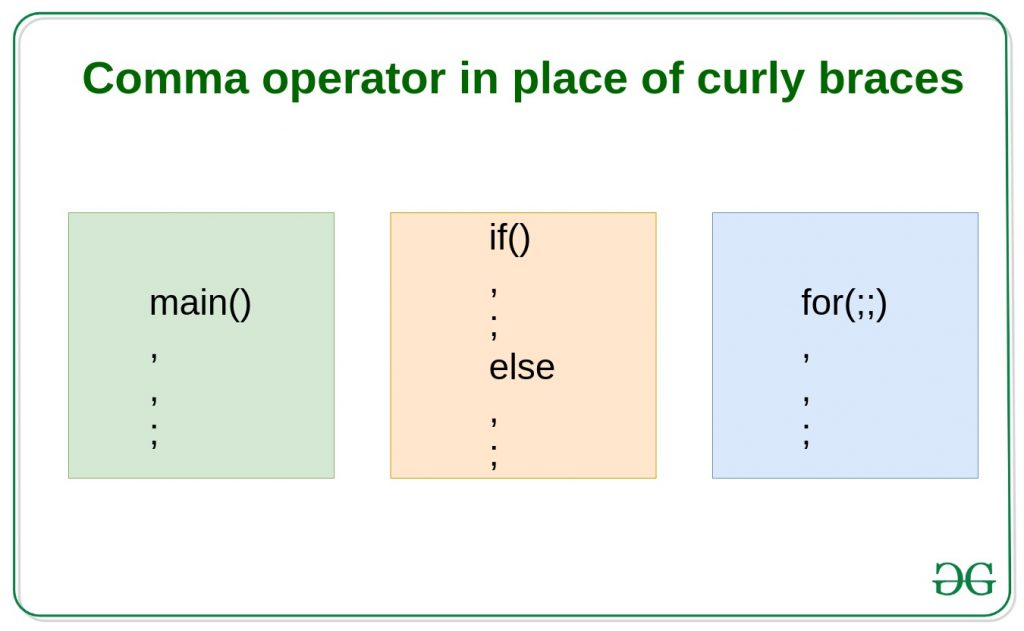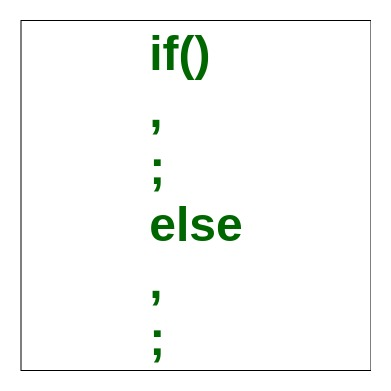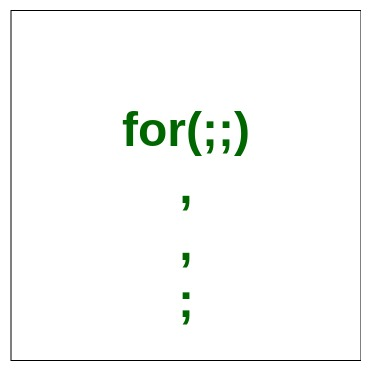# How can we use Comma operator in place of curly braces?

In C and C++, comma (, ) can be used in two contexts:

But in this article, we will discuss how a comma can be used as curly braces.

The curly braces are used to define the body of function and scope of control statements. The opening curly brace ({) indicates starting scope and closing curly brace (}) indicates the end of the scope. It is also possible to use a comma operator in control statements to define scope instead of using curly braces.### Comma operator used in place of curly braces in if-else statements:Example:

 `// C++ program to show how to use ` `// comma in place of curly braces ` `// for if-else statements ` ` `  `#include ` `using` `namespace` `std; ` ` `  `void` `func(``int` `num) ` `{ ` `    ``if` `(num < 10) ` `        ``cout << ``"It shows how we can use "` `             ``<< ``"comma operator in place of curly braces.\n"``, ` `            ``cout << ``"Entered number is less than 10\n"``, ` `            ``cout << ``"end of if block is encountered\n\n"``; ` `    ``else` `        ``cout << ``"Now we are in else part\n"``, ` `            ``cout << ``"Entered number is greater than 10\n"``, ` `            ``cout << ``"End of else is encountered\n\n"``; ` `} ` ` `  `int` `main() ` `{ ` `    ``int` `num = 5; ` `    ``func(num); ` ` `  `    ``num = 20; ` `    ``func(num); ` ` `  `    ``return` `0; ` `} `

Output:

```It shows how we can use comma operator in place of curly braces.
Entered number is less than 10
end of if block is encountered

Now we are in else part
Entered number is greater than 10
End of else is encountered
```

### Comma operator used in place of curly braces in loops:Example:

 `// C++ program to show how to use ` `// comma in place of curly braces ` `// for loops ` ` `  `#include ` `using` `namespace` `std; ` ` `  `void` `func(``int` `num) ` `{ ` `    ``for` `(``int` `i = 0; i < num; i++) ` `        ``cout << ``"It shows how we can use "``, ` `            ``cout << ``"comma operator in place of curly braces.\n"``, ` `            ``cout << ``"Loop traversal number: "``, ` `            ``cout << i << ``"\n\n "``; ` `} ` ` `  `int` `main() ` `{ ` `    ``int` `num = 5; ` `    ``func(num); ` ` `  `    ``return` `0; ` `} `

Output:

```It shows how we can use comma operator in place of curly braces.
Loop traversal number: 0

It shows how we can use comma operator in place of curly braces.
Loop traversal number: 1

It shows how we can use comma operator in place of curly braces.
Loop traversal number: 2

It shows how we can use comma operator in place of curly braces.
Loop traversal number: 3

It shows how we can use comma operator in place of curly braces.
Loop traversal number: 4
```

Note: The last statement of the block must be terminated by a semicolon.

Attention reader! Don’t stop learning now. Get hold of all the important DSA concepts with the DSA Self Paced Course at a student-friendly price and become industry ready.

My Personal Notes arrow_drop_upCheck out this Author's contributed articles.

If you like GeeksforGeeks and would like to contribute, you can also write an article using contribute.geeksforgeeks.org or mail your article to contribute@geeksforgeeks.org. See your article appearing on the GeeksforGeeks main page and help other Geeks.

Please Improve this article if you find anything incorrect by clicking on the "Improve Article" button below.

Article Tags :
Practice Tags :

15

Please write to us at contribute@geeksforgeeks.org to report any issue with the above content.